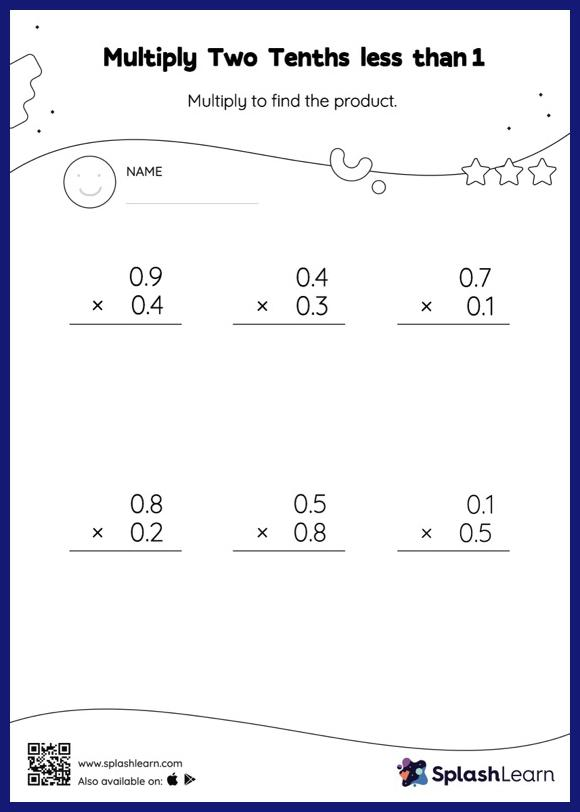# Multiply Two Tenths less than 1: Vertical Multiplication Worksheet

Home > Multiply Two Tenths less than 1: Vertical MultiplicationCan your young mathematician multiply two tenths less than 1? That's exactly what they do here. The product of a tenth multiplied by another tenth will have two decimal digits. In the multiply two tenths less than 1 worksheet, students practice this idea by multiplying the numbers without considering the decimal points and then adding the decimal point two places from the right in the product.